## Abel's Identity

Given a homogeneous linear Second-Order Ordinary Differential Equation,(1)

call the two linearly independent solutionsand. Then(2)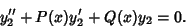(3)

Now, take(3) minus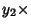(2),(4)(5)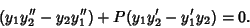(6)

Now, use the definition of the Wronskian and take its Derivative,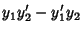(7)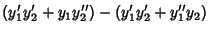(8)

Plugging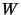and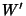into (6) gives(9)

This can be rearranged to yield(10)

which can then be directly integrated to(11)

whereis the Natural Logarithm. Exponentiation then yields Abel's identity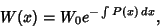(12)

where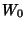is a constant of integration.